﻿ Random Number Program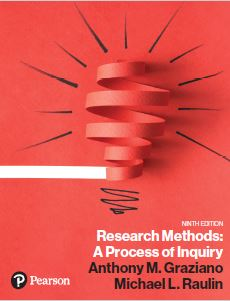Graziano & Raulin
Research Methods (9th edition)

## Random Number Generator Program

### Graziano and Raulin

Many of the critical control procedures in research design involve using random numbers. Appendix B includes a list of random numbers, with instructions of how to use that list for randomization. However, it is often easier to simply use a program for generating random numbers. There is such a program online to generate lists of random numbers. You can access that program by using the random.org link.

Operational Instructions

Random numbers tend to be used for several different tasks. We will list those tasks and show you how to achieve each of those goals using the random.org program. Clicking on this link will open the following site.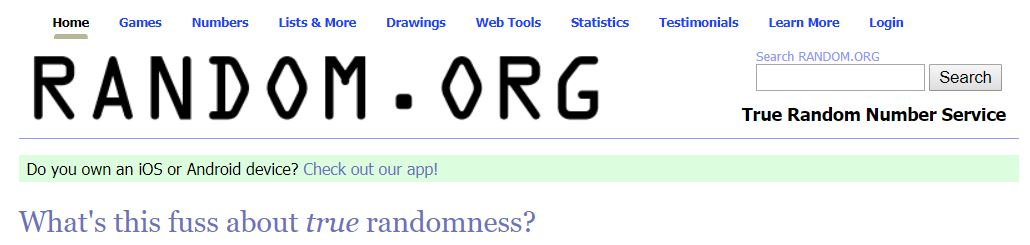Simple List of Random Numbers

This option will give you a list of random numbers with no constraints on the numbers. The program will ask you for the highest number desired, the lowest number desired, and how many random numbers you want. This option will effectively produce a random number table such as the one that appears in Appendix B of the text. In fact, a program like this one created the random number table that appears in the text.

To create a list of random numbers:

1.    Go to Random.org (see above).

2.    Click on Numbers and then Integers from the drop-down menu

3.    Complete the form to get the results that you want. For example, in the form shown below, you can see that we set up the program to give us 10,000 integers from 0 to 9 and to print them out in 20 columns.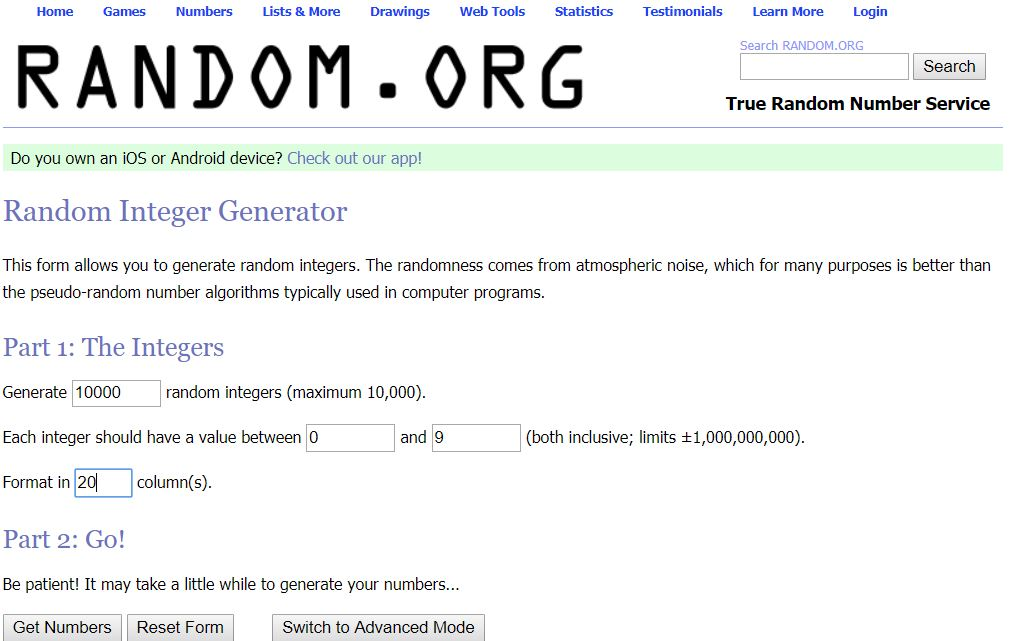List of Random Numbers with No Repeated Numbers

This option will give you a list of random numbers with no duplicate numbers. This is helpful if you are randomly sampling from a population in which every individual in the population is numbered from 1 to N (the total population size). The program will ask you for the highest number desired and the lowest number desired. It will print the randomized sequence for the entire population. For example, if you want to sample 50 subjects from a population of 4000, number each subject in the population from 1 to 4000 and then request that the program give a sequence between 1 and 4000. Then just take the first 50 numbers and ignore the remaining 3950.

To create a list of unduplicated numbers:

1.    Go to Random.org.

2.    Click on Numbers and then Sequences in the drop-down menu.

3.    Complete the form shown below. We have set it up to sample 50 people from a population of 400. We assume that each of the members of the population have a unique number assign to them starting with 1 and continuing to 400. So we set the boundaries for the sequence as 1 to 400 and format for convenience the output in 10 columns.

4.    When we click on the button that says get sequence, we will get the 400 numbers in a random order. Since we only need 50 subjects, we simply copy the first five rows to identify which subjects to use.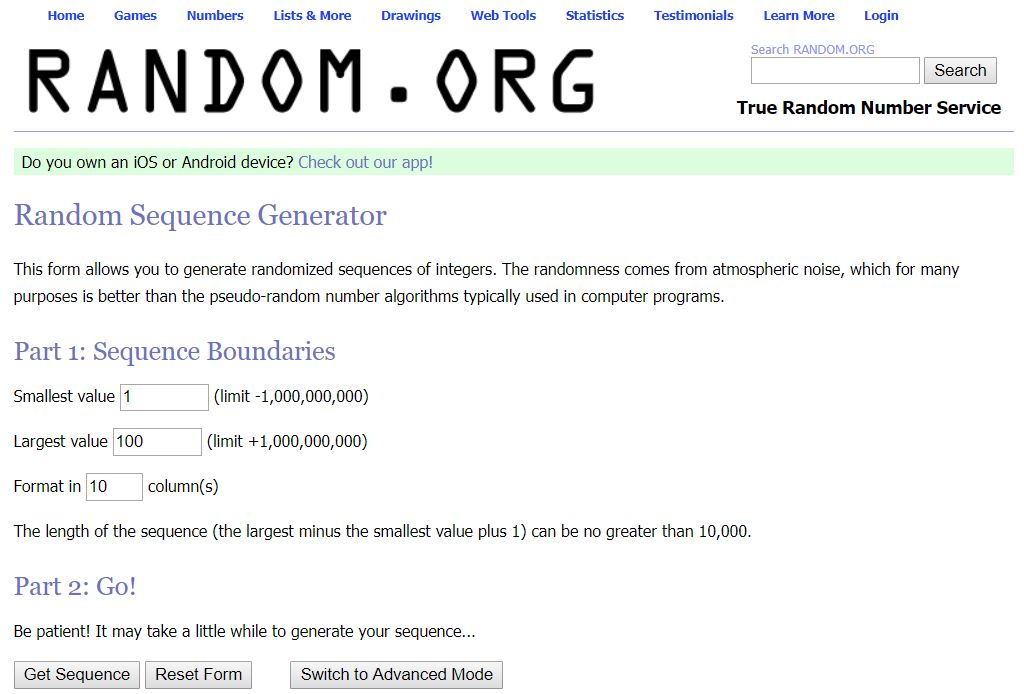Free Random Assignment to Groups

This option will produce a list of random numbers for random assignment to groups. There are no restrictions on the random numbers, so it is likely that the groups will not have the same sample size. The program will ask you for the number of groups and the number of subjects that you want to assign.

To create a free random assignment list:

1.    Go to random.org.

2.    Click on numbers and then integers in the drop-down menu.

3.    In our example below, we wanted to randomly assign 100 subjects to one of three groups. So we asked the program to generate 100 random numbers between 1 and 3 and, for convenience, we had the program print those numbers in 10 columns.

4.    Clicking on Get Numbers will produce our random list.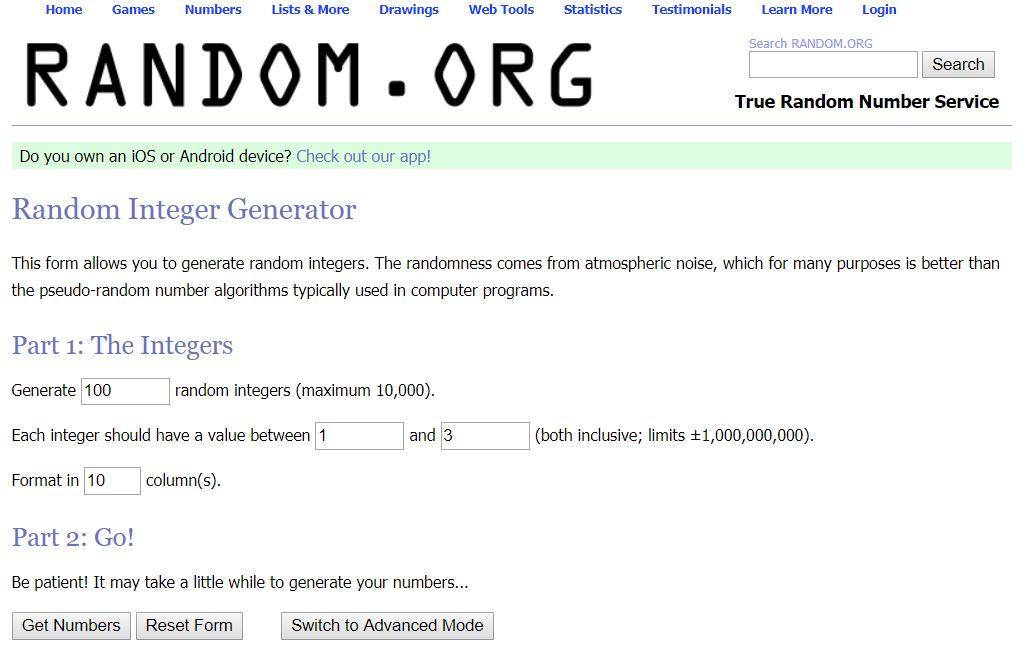Random Assignment within Blocks to Groups

This option will produce a list of random numbers for random assignment to groups in which the groups are randomized within blocks. For example, if you have four groups and 60 subjects to assign, the first four random numbers will be 1 through 4 in a random order, followed by another random order of 1 through 4, and so on.

Randomizing within blocks assures that the number of subjects will be equal or close to equal in the groups. It is also useful for matched random assignment or for generating random ordering of conditions for within-subjects designs.

To create a randomized within blocks list of random numbers:

1.    Go to Random.org.

2.    Click on Numbers and then Integer sets in the drop-down menu

3.    In our example, we assume that there are five conditions and that we intend to run 50 subjects. Therefore, we have to create 5 blocks of conditions (5 blocks timed 10 sets equals 50 subjects). We request that the integers be between 1 and 5 (each number representing one of the groups).

4.    It is VERY important in step 2 to UNCLICK the “sort the members of each set in ascending order.” If you do not do this and you run the conditions specified above, you will get 10 sets that will read 1, 2, 3, 4, 5.

5.    Click on Get Sets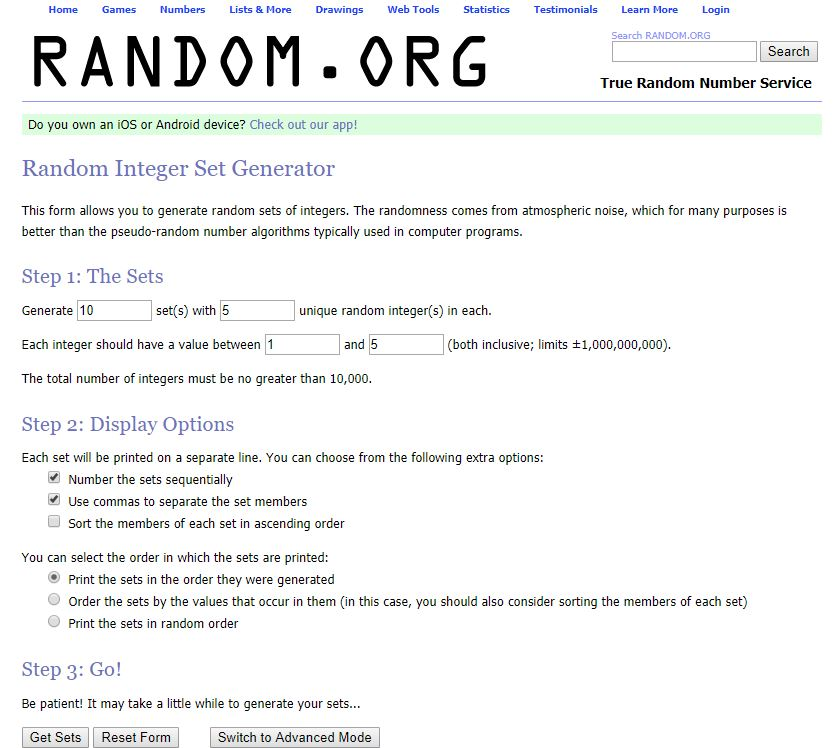Note that when using the program for random ordering of conditions or for matched-random assignment, the procedure varies slightly.

·         For random ordering of conditions, the number of sets is the number of subjects that you intend to use. If there are three conditions and you intend to run 60 subjects, you would generate 60 sets with 3 unique random integers. The integers should be between 1 and 3. Again, make sure that you have unchecked “sort the members of each set in ascending order.”

·         For matched random assignment, the number of sets is the number of matched sets. For example, if we have 50 matched pairs (100 subjects total) and we want to assign each member of the pair randomly to two groups, we would ask for 50 sets of two unique integers and set the integer range from 1 to 2. Again, make sure that you have unchecked “sort the members of each set in ascending order.” If the set says 1, 2, then the first subject in the pair goes in group 1 and the second subject in group 2. If the set says 2, 1, we do the opposite (first subject in groups 2 and the second subject in group 1).